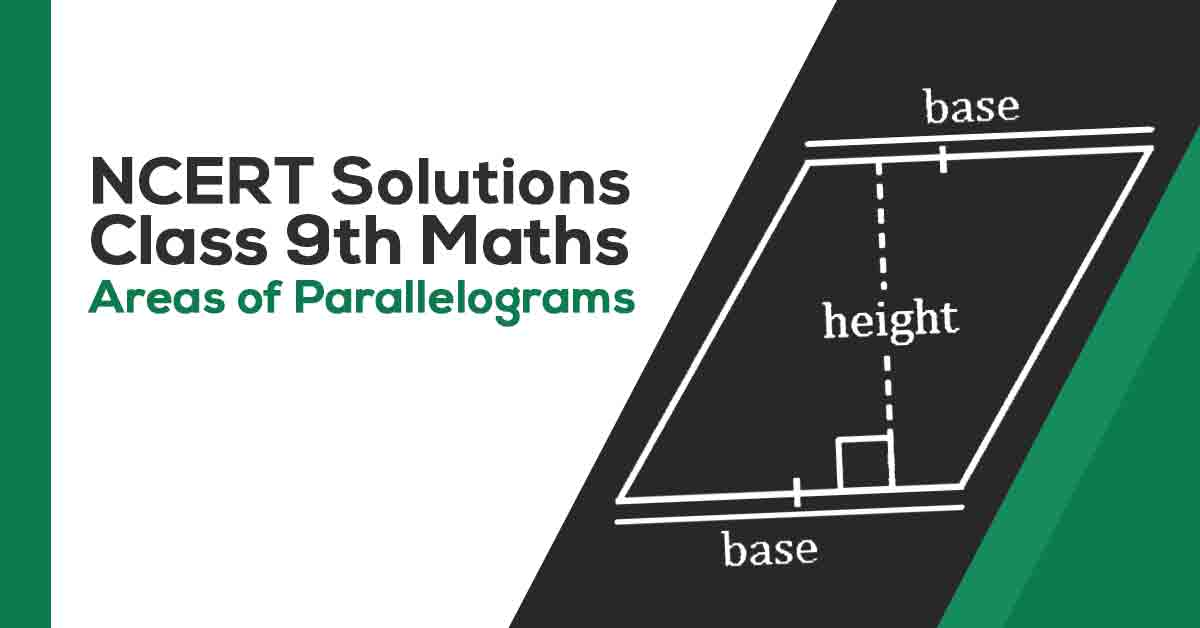NCERT Solutions for Class 9 Maths Chapter 9 | Vidyakul
×

# NCERT Solutions For Class 9 Maths Chapter 9

## NCERT Solutions Class 9 Maths Chapter 9 Area of Parallelograms and Triangles - PDF DownloadStudents can take help from NCERT Solutions for Class 9 Maths Class 9 Area of Parallelograms and Triangles to study for their examinations. In this chapter, you will learn that Area of a figure is a number (in some unit) associated with the part of the plane enclosed by that figure. Two congruent figures have equal areas but the converse need not be true.

These CBSE NCERT Solutions for Class 9 Maths Chapter 9 Area of Parallelograms and Triangles will help you to practice all the topics in the chapter with ease and score maximum marks in the examination.